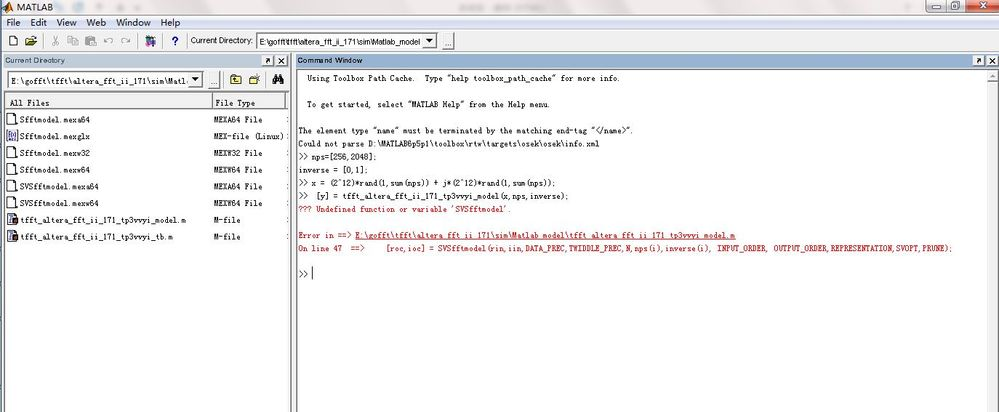Community
cancel
Showing results for
Did you mean:Novice
160 Views

## Intel FFT simulation was failed in 2 Matlab version

Quartus prime pro 17.1 + Modelsim 10.5c +Arria10gx

Intel FFT IP core , Variable Streaming,N=256,data type is single.

I generated an Intel FFT ipcore from Quartus, I can see some Matlab   <variation name>_model and <variation name>_tb files have been generated under my nominated location E:\gofft\tfft\altera_fft_ii_171\sim\Matlab_model\

Following the simulation instruction, 2.5.2. Simulating the Variable Streaming FFT IP Core in the MATLAB, on page 24 of ug_fft.pdf. I got something wrong as below

>> nps=[256,2048];

inverse = [0,1];

>> x = (2^12)*rand(1,sum(nps)) + j*(2^12)*rand(1,sum(nps));

>> [y] = tfft_altera_fft_ii_171_tp3vvyi_model(x,nps,inverse);

Invalid MEX-file

denied.

Error in tfft_altera_fft_ii_171_tp3vvyi_model (line 47)

[roc,ioc] = SVSfftmodel(rin,iin,DATA_PREC,TWIDDLE_PREC,N,nps(i),inverse(i), INPUT_ORDER,

OUTPUT_ORDER,REPRESENTATION,SVOPT,PRUNE);

Because I have noticed some old answer for similar question mentioned this simulation only support 32bit Matlab. So I have tried this test on below 2 platform,

Both 2 platform,I got same error information .I have restart matlab and computer ,and issue still same.

Cast case 1 :Win10 + MatlabR2019b

Cast case 2 :Win7+ Matlab6.5.1

Any suggestion is welcome

Thanks

Jim

Cast case 1 :Win10 + MatlabR2019bCast case 2 :Win7+ Matlab6.5.14 RepliesNovice
103 Views

my matlab files generated by IP tools , you can try it on you own platform to see if you can duplicate my issue.Employee
103 Views

Hi,

As I understand it, you encounter some issue when trying to simulate the Matlab model generated together with the IP HDL generation. For your information, can you try the following:

1. In your FFT IP, Generate the Example Design

2. In the generated folder ie fft1_ii_0_example_design, look for Matlab_model folder

3. You can find the model.m and _tb.m in the folder

4. Try run the Matlab simulation using these files.

Please let me know if there is any concern. Thank you.

Best regards,

Chee PinNovice
103 Views

Hi Chee pin,

Unfortunately, there is no option to generate a example as below.Employee
103 Views

Hi,

thanks for your update. As I further debug into this, I notice that there is no example design generation for A10 FFT in QPro. As a workaround, you can try to use Quartus Standard Edition to generate the example design. I have emailed you a ZIP of the example design generated with Q17.0Std for your reference. Sorry for the inconvenience.